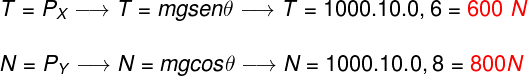Mechanics

# Newton’s Laws

Newton’s laws are a set of laws that describe the dynamics of motion. Newton’s first law, known as the law of inertia , deals with resistance to change in state of motion; Newton’s second law , known as the fundamental principle of dynamics, addresses the definition of resultant force and its relationship with acceleration; finally, Newton’s third law , the law of action and reaction, describes the pairs of forces that arise from the interaction between bodies.

## Introduction to Newton’s Laws

Newton’s laws were published in 1687 by the English physicist Isaac Newton . His main work, entitled Mathematical Principles of Natural Philosophy, laid the foundations of dynamics and was able to explain the emergence of tides and planetary orbits, for example.

### Force, acceleration and resultant force

Force , acceleration and resultant force are fundamental concepts to understand Newton’s laws.

So, what is a force? Force is a vector physical quantity , measured in the unit of kg.m/s², or N (newton), capable of changing the state of motion of a body. In other words, when two bodies exert forces on each other, their states of motion can change, this implies that the application of forces on a body can result in the emergence of an acceleration, the concept that will be discussed next.

Acceleration is the change in speed. Every body that has mass opposes the appearance of an acceleration, this property inherent in matter is called inertia. According to Newton’s laws, if a non-zero resultant force is acting on a body, it will be subject to an acceleration.

Resulting force is obtained based on the vector sum of all forces acting on a body. Because they are vectors, the forces add up and can also cancel each other out. The result of the vector sum of these forces gives rise to the resultant force.

Newton’s 1st law: law of inertia

Newton’s first law states that every body has the tendency to remain at rest or in rectilinear and uniform motion , if the resultant of the forces acting on it is zero. This law indicates that a stationary body or one moving with constant velocity is in equilibrium, that is, even if thousands of forces act on it, they cancel each other out.

An example of a situation in which the net force is zero is the motion of a body in a space vacuum , in a region of zero gravity . Once launched in motion, this body will tend to move forever in a straight line, unless a force changes its speed or direction.

“Every body remains at rest or in uniform motion along a straight line unless it is forced to change its state by forces applied to it.”

## Newton’s 2nd law: fundamental principle of dynamics

Newton’s second law concerns acceleration. According to this law, if a body is subjected to a non-zero resultant force, it will present an acceleration in the direction of that resultant force. The formulation of Newton’s second law is based on an equation, note: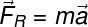R – resultant force (N)

m – mass (kg)

a – acceleration (m/s²)

Originally, Newton’s second law was written in terms of a physical quantity called momentum or linear motion. According to the statement of this law, the resultant force on a body is determined by the variation of its momentum with respect to an interval of time. Check this equation: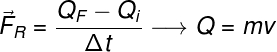F and Q i – final and initial momentum (kg.m/s)

Δt – time interval (s)

v – speed (m/s)

The equation shown is also used to define what impulse is . According to Newton’s second law, impulse is the change in momentum, this change arises from the application of a force during a certain time interval, thus: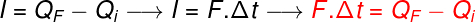I – impulse (kg.m/s)

## Newton’s 3rd law: law of action and reaction

Newton’s third law explains that if body A applies a force to body B, body B will produce a reaction force on A. Action and reaction forces always have the same magnitude and act in the same direction, however, they point in opposite directions. According to this law, forces arise in pairs and it is not possible for a pair of action and reaction forces to arise in a single body.

Several situations illustrate the operation of Newton’s third law, for example:

• To walk, we push the ground back, the ground, in turn, pushes us forward, due to the friction force established between our feet and the ground.
• To move on water, the blades of a boat’s propellers push the water backwards, and the water, in turn, pushes the boat forward.

## Applications of Newton’s Laws

Newton’s laws can be applied to several situations, among the most important are the friction forces , the decomposition of weight on the inclined plane and the application of centripetal forces in curved paths.

### strength weight

The weight force is the force of attraction exerted by gravity The weight of a body is calculated by the product of its mass and gravitational acceleration.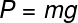P – weight (N)

m – mass (kg)

g – gravity (m/s²)

### Frictional force

The friction force exists because no surface is perfectly smooth. Microscopically, the relief of surfaces, even the smoothest, is uneven. There are two friction situations: static friction and kinetic friction, for each of these situations, we use different coefficients of friction. The figure below shows the formula used to calculate this force, check it out: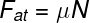at – friction force (N)

μ – coefficient of friction

N – normal force (N)

### inclined plane

Bodies resting on inclined surfaces have their weight force divided into components. These components, called the horizontal component (PX) and vertical component (PY), can be calculated by decomposing the force-weight vector, check out how: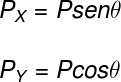X – horizontal component of the weight (N)

Y – vertical component of weight (N)

θ – angle between the ramp and the horizontal surface (º)

### Centripetal force

Centripetal force is the net force on a body moving along a circular path. The centripetal force always points towards the center of a curve, and can be calculated by means of the vector sum of the forces that point towards the radius of the curve.

Unlike what we usually hear, centrifugal force does not exist, because it is not a force, but the inertia of the body in action. It’s just like when we spin a bucket full of water. The reason the water inside the bucket doesn’t fall is the direction of the net force: at the highest point, the centripetal force points downwards, so the water tends to oppose movement in that direction.

A similar case is that of the ball hanging from a string attached to the roof of a bus. If the bus accelerates to the right, the ball tends to remain on the left, and vice versa.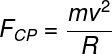CP – centripetal force (N)

m – mass (kg)

v – speed (m/s)

R – radius of the curve (m)

## Newton’s Laws and Gravity

When applied to the context of gravitation, Newton’s laws gave rise to the theory of universal gravitation . According to this theory, the gravitational force of attraction is proportional to the product of the masses that attract each other but also inversely proportional to the distance that separates them. Check the formula used to calculate the gravitational force: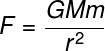G – universal gravitation constant (6.67.10 -11 Nm²/kg²)

M in – masses of bodies (kg)

r – distance between bodies (m)

Based on the law of universal gravitation, it was possible to determine the orbit of different celestial bodies such as planets and asteroids . Furthermore, through it, it is possible to obtain the results described by Kepler’s laws , which deal with planetary and satellite orbits .
Exercises on Newton’s Laws

(Enem 2017) In a frontal collision between two cars, the force that the seat belt exerts on the driver’s chest and abdomen can cause serious damage to internal organs. Thinking about the safety of its product, a car manufacturer carried out tests on five different models of belt. The tests simulated a collision lasting 0.30 seconds, and the dolls representing the occupants were equipped with accelerometers. These devices record the doll’s deceleration module as a function of time. Parameters such as mass of the dolls, dimensions of the belts and speed immediately before and after impact were the same for all tests. The final result obtained is in the graph of acceleration by time.

Which belt model offers the lowest risk of internal injury to the driver?

to 1

b) 2

c) 3

d) 4

e) 5

feedback:

Resolution: Letter B

Analyzing the graph, it is possible to see that the belt that provides the smallest deceleration amplitude is number 2.

(Enem 2018)In cartoons it is common to see the character trying to propel a boat by blowing air against the sail to compensate for the lack of wind. Sometimes it uses its own breath, bellows or fans. Students from a teaching laboratory decided to investigate this possibility. For this, they used two small plastic cars, A and B, installed on these small fans and vertically fixed a parabolic curvature card to perform a function analogous to the sail of a boat. In car B, the direction of the fan was reversed and the sail was maintained, in order to maintain the characteristics of the boat, mass and shape of the cardboard. The figures represent the cars produced. Car assembly A seeks to simulate the cartoon situation, as the fan is directed towards the sail.

ith the cars oriented according to the figures, the students turned on the fans, waited for the airflow to become steady, and determined the modulus of the average speeds of cars A (V A ) and B (V B ) for the same time interval.

Regarding the intensities of the average speeds and the direction of motion of car A, the students observed that:

a) V A = 0; VB > 0 ; car A does not move.

b) 0 < V A < V B ; car A moves to the right.

c) 0 < V A < V B ; car A moves to the left.

d) 0 < V B < V A ; car A moves to the right.

e) 0 < V B < V A ; car A moves to the left.

feedback :

Resolution: Letter B

Although the official test template suggests alternative A, the correct answer is letter B. Several studies show that the boat, sail and air system does not constitute a closed system of bodies, in which the resultant force must be zero. Thus, the principle of conservation of momentum cannot be applied to this case. What actually happens is that the inelastic collision between the air particles and the sail moves the boat in the same direction as the incident wind.

(Unigranrio – Medicina – 2017) To keep a car of mass 1000 kg on a smooth inclined ramp that forms an angle θ with the horizontal, a cable is attached to it. Knowing that the car, under these conditions, is at rest on the inclined ramp, check the option that indicates the intensity of the normal reaction force of the ramp on the car and the tension in the cable that supports the car, respectively. Despise friction. Data: sin θ = 0.6 cos θ = 0.8 and g = 10 m/s².

a) 8000 N and 6000 N

b) 6000 N and 8000 N

c) 800 N and 600 N

d) 600 N and 800 N

e) 480 N and 200 N

Template: Letter A

Resolution:

In order for the cart to stand still, it is necessary that the traction applied to it is of an intensity equal to the x component of its weight, similarly, the normal force must have the same magnitude as the vertical component of the cart’s weight, check: